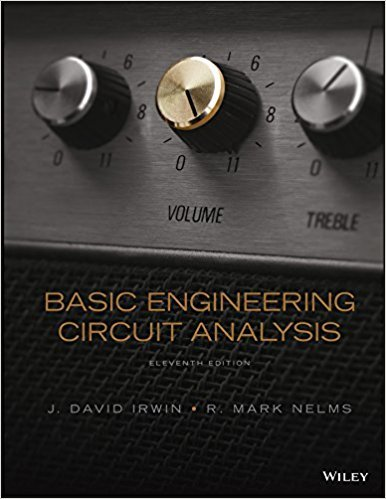×
Get Full Access to Basic Engineering Circuit Analysis - 11 Edition - Chapter 6 - Problem 6.5
Get Full Access to Basic Engineering Circuit Analysis - 11 Edition - Chapter 6 - Problem 6.5

×

# The voltage across a 50-F capacitor is shown in Fig. P6.5.ISBN: 9781118539293 159

## Solution for problem 6.5 Chapter 6

Basic Engineering Circuit Analysis | 11th Edition

• Textbook Solutions
• 2901 Step-by-step solutions solved by professors and subject experts
• Get 24/7 help from StudySoup virtual teaching assistantsBasic Engineering Circuit Analysis | 11th Edition

4 5 1 390 Reviews
14
2
Problem 6.5

The voltage across a 50-F capacitor is shown in Fig. P6.5. Determine the current waveform. 0 246 8 10 10 10 12 t (ms) (t) (V) Figure P6.5

Step-by-Step Solution:
Step 1 of 3

PHY 184 Week 7 Notes-DC Circuits 10/10-10/13  Most practical circuits cannon be resolved into series or parallel systems of capacitors and resistors o To handle this we use Kirchhoff’s rules  A Junction is a place where 3+ wires connect o Each connection between 2 junctions is called a branch  Each branch has the same current within the branch  A Loop in a current is any set of connected wires forming a closed path o You can get back to where you started Kirchhoff’s Junction Rule  The sum of currents entering a junction equals the sum of currents leaving the junction  Positive terms enter, negative terms exit n i =0 o k=1k  Consider a Junction with current i e1tering and i an2 i lea3ing

Step 2 of 3

Step 3 of 3

##### ISBN: 9781118539293

Unlock Textbook Solution